# SSAT Middle Level Math : How to subtract variables

## Example Questions

← Previous 1 3 4 5

### Example Question #1 : How To Subtract Variables

Simplify: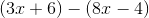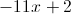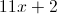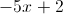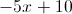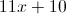Explanation: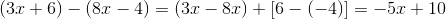### Example Question #2 : How To Subtract Variables

Simplify: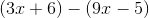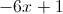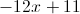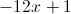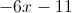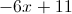Explanation: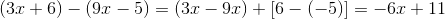### Example Question #2 : How To Subtract Variables

Simplify: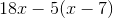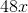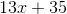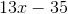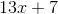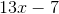Explanation: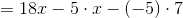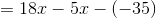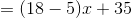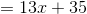### Example Question #4 : How To Subtract Variables

Simplify: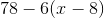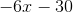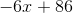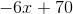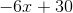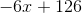Explanation: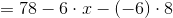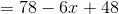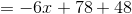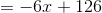### Example Question #2 : How To Subtract Variables

Solve for: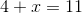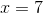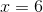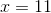Explanation:

In order to solve for, moveto one side of the equation and everything else to the other. To do this, subtractfrom both sides.

### Example Question #6 : How To Subtract Variables

Simplify: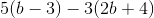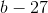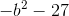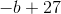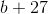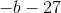Explanation:

The first step is to apply the distributive property. Don't forget to distribute the negative in the second parenthesis!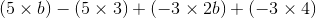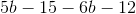Next, combine the variables and the numbers. This gives us: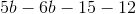### Example Question #4 : How To Subtract Variables

Which of the following phrases can be written as the algebraic expression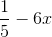?

One fifth the product of negative six and a number

None of the other responses is correct.

Six less than one fifth of a number

One fifth less than the product of six and a number

One fifth decreased by the product of six and a number

One fifth decreased by the product of six and a number

Explanation:is one fifth decreased by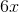is the product of six and a number.

Consequently,is "one fifth decreased by the product of six and a number".

### Example Question #5 : How To Subtract Variables

Which of the following phrases can be written as the algebraic expression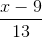?

The correct answer is not among the other choices.

Thirteen divided into the difference of nine and a number.

Thirteen divided into the difference of a number and nine.

Thirteen divided by the difference of a number and nine.

Thirteen divided by the difference of nine and a number.

Thirteen divided into the difference of a number and nine.

Explanation:is thirteen divided into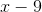.is the difference of a number and nine.

Therefore,is "thirteen divided into the difference of a number and nine".

### Example Question #1 : Expressions & Equations

Simplify: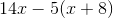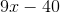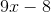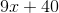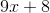Explanation: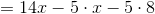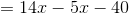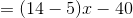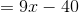### Example Question #3 : How To Subtract Variables

Simplify: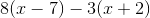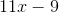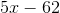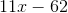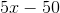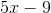Explanation: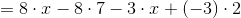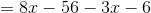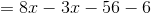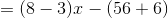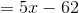← Previous 1 3 4 5